# LCD Display Inverter

Display Inverter / VGA Board / LCD Controller

## Three compact solutions for high step-down ratios

【Introduction】This article will explain why non-isolated DC-DC step-down converters (referred to in this article as buck converters) face serious challenges in converting high DC input voltages to very low output voltages at high output currents . This article will describe three different ways to achieve a high step-down ratio while maintaining a small size.

question:

What are some ways to achieve a compact design at a high step-down ratio?

This article will explain why non-isolated DC-DC step-down converters (referred to as buck converters in this article) face serious challenges when converting high DC input voltages to very low output voltages at high output currents. This article will describe three different ways to achieve a high step-down ratio while maintaining a small size.

Introduction

System designers may face the challenge of down-converting high DC input voltages to very low output voltages (such as from 60 V to 3.3 V at 3.5 A) at high output currents while maintaining high system efficiency and small size And achieve a simple design.

Combining high input-output voltage differential with high current automatically excludes linear regulators due to excessive power dissipation. Therefore, the designer must choose the switching topology under these conditions. However, even with this topology, it is still quite difficult to achieve a sufficiently compact design for space-constrained applications.

Challenges for DC-DC Buck Converters

To achieve a high step-down ratio, one option is to use a buck converter, as it efficiently reduces the input voltage to a lower output voltage (for example, VIN = 12 V down to VOUT = 3.3 V), still have a lot of current, and maintain a small size as a topology option. However, in some cases, buck converters face serious challenges in maintaining a stable output voltage. To understand these challenges, we need to remember that the duty cycle (D) of a buck converter operating in continuous conduction mode (CCM) can be simplified to: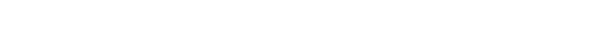duty cycle and switching frequency (fSW) is as follows, where the on-time (tON) is the length of time the control FET remains on during each switching period (T):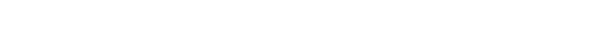Combining Equation 1 and Equation 2, it can be seen that tONHow is affected by the step-down ratio and fSWImpact: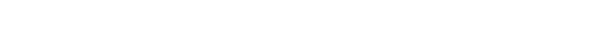It can be seen from Equation 3 that when the input-output voltage ratio (VIN⁄VOUT) and ⁄ or fSWWhen increased, the on-time decreases. This means that the buck converter must be able to operate with a very low on-time to operate at high VIN⁄VOUTratio regulates the output voltage in the CCM while at high fSWThis will be more difficult to achieve.

We assume that in an application, VIN(MAX) = 60 V, VOUT = 3.3 V, IOUT(MAX) = 3.5A. Where necessary, we need to use the values ​​in the LT8641 data sheet, as in the following chapters we will provide a solution using the LT8641. Required minimum on-time (tON(MIN)) corresponds to the highest input voltage (VIN(MAX)). To evaluate this tON(MIN), it is suggested to improve the accuracy of Equation 3. Voltage drop V across the two power MOSFETs containing the buck converterSW(BOT)and VSW(TOP)and use VIN(MAX)Alternative VINwe get: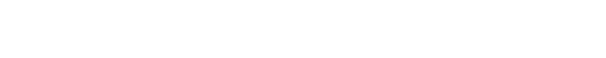By using V in Equation 4IN(MAX)fSW = 1 MHz, we get tON(MIN)is 61 ns. To calculate VSW(BOT)and VSW(TOP)we used the R provided in the LT8641 data sheetDS(ON)(BOT) and RDS(ON)(TOP)value, and it is known that VSW(BOT) =RDS(ON)(BOT) × IOUT(MAX)VSW(TOP) =RDS(ON)(TOP) × IOUT(MAX). The value of 61 ns can be obtained from the above formula, such a short time value, it is difficult for the buck converter to guarantee tON(MIN); therefore, system designers have to look for alternative topologies. There are currently three possible solutions that can achieve high step-down ratios.

The value of 61 ns can be obtained from the above formula, such a short time value, it is difficult for the buck converter to guarantee tON(MIN); therefore, system designers have to look for alternative topologies. There are currently three possible solutions that can achieve high step-down ratios.

Three compact solutions, VIN(MAX) = 60 V, VOUT = 3.3 V, IOUT(MAX) = 3.5A

Solution 1: Use LT3748 Non-Optocoupler Flyback Transformer

The first option is to use an isolated topology, where the transformer has an N:1 turns ratio and is responsible for doing most of the downconversion. To this end, Analog Devices offers flyback controllers, such as the LT3748, which do not require a third transformer winding or opto-isolator, making the design simpler and more compact. Figure 1 shows an LT3748 solution suitable for this situation.

Although the LT3748 solution simplifies the design and saves space compared to standard flyback designs, it still requires the use of a transformer. For applications that do not require isolation of the input and output, it is best to avoid this component, which increases design complexity and size compared to non-isolated solutions.

Solution 2: Use LTM8073 and LTM4624 µModule Devices

As an alternative, designers can downconvert in two steps. To achieve a lower component count (only 10), 2 µModule® devices and 8 external components can be used, as shown in Figure 2. In addition, the two µModule devices have integrated their own power inductors, freeing the system engineer from a difficult design task. Both the LTM8073 and LTM4624 are available in BGA packages with dimensions of 9 mm × 6.25 mm × 3.32 mm and 6.25 mm × 6.25 mm × 5.01 mm (L × W × H), respectively, providing a small form factor solution.

Since the LTM4624 exhibits an efficiency of 89% under these conditions, the LTM8073 delivers up to 1.1 A to the input of the LTM4624. Since the LTM8073 can deliver up to 3 A of output current, it can be used to power other supply rails. For this, in Figure 2, we choose 12 V as the intermediate voltage (VINT).

Although transformers should be avoided, some designers may be reluctant to use a solution that requires two separate buck converters, especially if no intermediate voltage is required to power the other rails.

Solution 3: Use the LT8641 Buck Converter

Therefore, in many cases, using a single buck converter is the preferred solution, as it is the ideal solution with high system efficiency, small size, and simple design. However, we did not show earlier that buck converters cannot handle high VIN⁄VOUTand high fSW?

This statement may apply to most buck converters, but not all. ADI’s portfolio includes buck converters such as the LT8641, which have a short minimum on-time of 35 ns typically (50 ns maximum) over the entire operating temperature range. These specifications are all below the previously calculated minimum on-time of 61 ns, giving us a third feasible compact solution. Figure 3 shows how simple the LT8641 circuit is.

It is also worth noting that the LT8641 solution is probably the most efficient of the 3 solutions. In fact, if efficiency has to be further optimized compared to Figure 3, we can reduce fSWand choose a larger Inductor size.

Although f can also be reduced by solution 2SW, but the integrated power inductor cannot flexibly improve the efficiency above a certain point. Furthermore, the use of two continuous downconversion stages has less negative impact on efficiency.

When using solution 1, the efficiency of the flyback design is very high due to the operation in boundary mode and the removal of all components in the non-optical feedback design. However, efficiency cannot be fully optimized due to the limited number of optional transformers, whereas solution 3 has a wide range of inductors to choose from.Figure 1. Circuit solution using the LT3748 to downconvert a 60 V input to a 3.3 V output.Figure 2. Circuit solution using the LTM8073 and LTM4624 to downconvert a 60 V input to a 3.3 V output.Figure 3. Circuit solution using the LT8641 to downconvert a 60 V input to a 3.3 V output.

Another way to check if the LT8641 meets the requirements

In most applications, the only adjustable parameter in Equation 4 is the switching frequency. Therefore, we re-transform Equation 4 to evaluate the maximum f allowed by the LT8641 under the given conditionsSW. So, we get Equation 5, which is also available on page 16 of the LT8641 data sheet.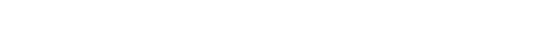We use this formula in the following example: VIN = 48 V, VOUT = 3.3 V, IOUT(MAX) = 1.5 A, fSW = 2 MHz. A 48 V input voltage is often used in automotive and industrial applications. Substituting these conditions into Equation 5, we get: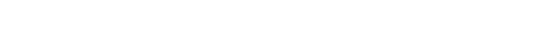Therefore, under the given application conditions, at fSWUp to 2.12 MHz, the LT8641 can operate safely, confirming that the LT8641 is a good choice for this application.

in conclusion

This paper presents three different approaches to achieve compact designs at high step-down ratios. The LT3748 flyback solution eliminates the need for bulky opto-isolators and is recommended for designs that require isolation of the input and output. The second approach requires the use of LTM8073 and LTM4624 µModule devices, a solution that can be useful when designers are hesitant to choose the best inductor for an application, and ⁄ or when an additional intermediate power rail must be provided. The third method, based on the LT8641 buck converter design, provides a compact and simple solution if only steep voltage downconversion is required.